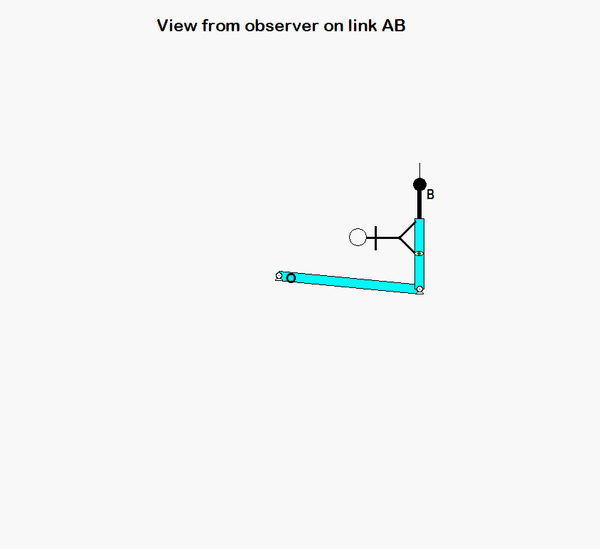# Homework H.3.ADiscussionAn animation from the simulation of the motion of the robotic arm system is shown above for a fixed observer. The motion of end-effector B is quite complicated, as can be seen. However, if we attach an observer to section AD of the end link of the arm, the observed motion of B is greatly simplified. In fact, the observer simply sees a back-and-forth motion of B in the x-direction, as shown in the animation below.HINTS: The velocity of point B can be found from the following moving reference frame velocity equation:

vB = vA + (vB/A)rel + ω × rB/A

vA = (L θ_dot) i
ω
= φ_dot k
(vB/A)rel = b_dot i

Use similar logic for writing down the acceleration of B. Be careful in writing down the expression for aA ; in particular, a0. (A is traveling on a circular path with a constant speed.)

## 9 thoughts on “Homework H.3.A”

1.Sung Namgung says:

How does the left figure and right figure different when I apply given values of parameters?

1.ecasetti says:

You don't need to worry about direction as much in the right figure because v_B will only be in the y direction since phi is 90 and theta is 0.

1.CMK says:

ecasetti: Be careful. I agree that two of the terms on the RHS of the equation for the velocity of B will be aligned with AD (the x-direction...straight up); however, the third term (the omega x r term) will be perpendicular to AD (in the y-direction...to the left). Does this make sense?

2.CMK says:

The left figure is for a generic position of the system. The right figure shows the system for the specific orientation of interest in this problem: θ = 0 and φ = 90 degrees.

2.hu665 says:

From the graph, it seems like the acceleration should have both negative i and j values, but I got a positive j since phi dot, v b/a and r b/a are all positive, what did I do wrong?

1.Qianyu Zhao says:

Remember the moving reference frame is defined 90 degrees apart from the fixed reference frame at this instant. Therefore, +i corresponds to +J and +j is on -I direction.

3.Sarah Nicole Lavelle says:

When finding the acceleration of A do we use the rigid body acceleration equation with point O?

4.Vincent Ricci says:

Does a_A equal to zero in the a_B equation? I'm not sure what acceleration could be when b_doubledot, theta_doubledot, and phi_doubledot are all equal to zero.

5.Vincent Ricci says:

If a_A and a_B/A both zero out, how do I calculate a_B with alpha as an unknown? Is it valid to assume that angular acceleration is in the same direction as angular velocity in this case?